Reinforcement learning (RL) encompasses a class of machine learning (ML) techniques that can be used to solve sequential decision-making problems. RL techniques have found widespread applications in numerous domains, including financial services, autonomous navigation, industrial control, and e-commerce. The objective of an RL problem is to train an agent that, given an observation from its environment, will choose the optimal action that maximizes cumulative reward. Solving a business problem with RL involves specifying the agent’s environment, the space of actions, the structure of observations, and the right reward function for the target business outcome. In policy-based RL methods, the outcome of model training is often a policy, which defines a probability distribution over the actions given an observation. The optimal policy will maximize the cumulative returns obtained by the agent.

In constrained decision-making problems, the agent is tasked with choosing the optimal actions under constraints. A distinct class of such problems exists wherein, depending on the state, the agent may be only allowed to choose from a subset of all actions. The remaining actions are inadmissible.

For example, consider an autonomous car that has 10 possible speed levels. This car may only be allowed to choose from a subset of its speed levels when traversing a residential neighborhood. Here, the constraint on the speed levels is determined by the location of the car. Such parameterized constraints on the actions are common in many real-world problems. Solving such problems with RL requires incorporating the constraints in the training process. Action masking is an approach to solve RL problems that involve inadmissibility constraints in a sample efficient manner. As the name suggests, it involves masking any inadmissible actions by setting their sampling probability to zero. The following figure depicts the RL cycle with action masking. It consists of an agent, the constraints that determine the action masks, the masks, state transitions, and the observed rewards.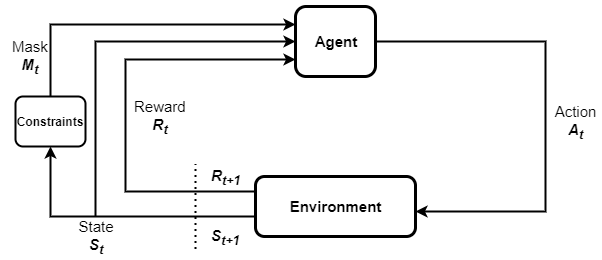In this post, we describe how to implement action masking with Amazon SageMaker RL using parametric action spaces in Ray RLlib. We describe an example problem that involves discrete multidimensional action spaces and multiple constraints. To access the complete notebook for this post, see the SageMaker notebook example on GitHub.

## Use case overview

We consider an example portfolio optimization problem in which an investor trades multiple asset types to maximize their total portfolio value. The portfolio consists of three different asset types, and a cash balance that simply refers to money you have in your bank account. During each investment period, the agent has to choose the quantity of each asset type that they buy or sell. The agent uses the available cash balance to finance any asset purchases. There are also transactions costs associated with each asset buy/sell action. The market price of each asset is assumed to vary across time. The prices are sampled randomly but modeled to show distinct behavior with different levels of volatility. The price ranges for the three asset classes are shown in the following figure.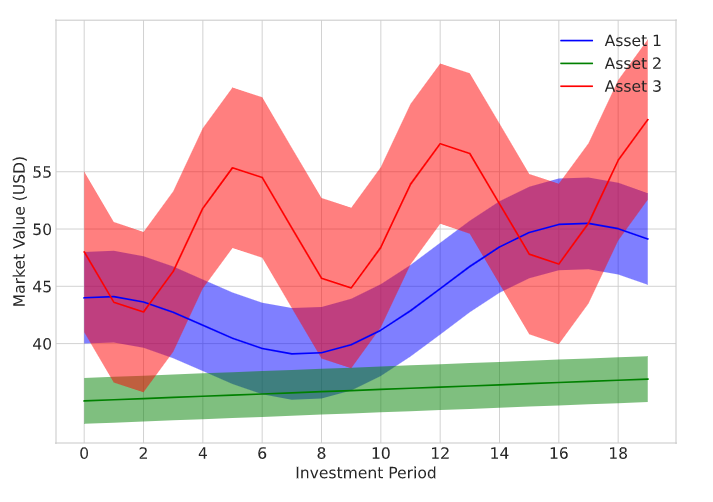The set of admissible actions for the agent are determined by parameters such as the current total portfolio value, current cash balance, the number of each types of assets held, and their current market value. For this problem, we enforce the following constraints on possible actions:

• C1 – The agent can’t sell more units of any asset type than what they currently own. For example, if the agent has 100 units of Asset 3 at time k in their portfolio, then it can’t sell 120 units of that asset at that time.
• C2 – Asset 3 is considered highly volatile by investors. The agent is not allowed to buy Asset 3 if the total value of their holdings in Asset 3 is above a third of their total portfolio value.
• C3 – Consumers of the RL model have a moderate risk preference and consider Asset 2 a conservative buy. As a result, the agent is not allowed to buy Asset 2 when the total value of Asset 2 holdings cross two-thirds of the total portfolio value.
• C4 – The agent can’t buy any assets if its current cash balance is less than \$1 USD.

## Set up the environment

To start, provision a SageMaker notebook instance via Amazon SageMaker Studio. For more information, see Use Amazon SageMaker Notebook instances.

Next, we implement the portfolio trading problem in a custom Open AI Gym environment and train an RL agent using SageMaker RL. A Gym environment provides an interface for the RL agent to interact with its environment, and to generate rewards and observations. The environment for the portfolio trading is located in the `trading.py` module. We use the `__init__` method to define and initialize some environment parameters. This includes transaction costs associated with asset buy/sell actions, mean value of the asset prices, price variances, and more. We also define the observation and action spaces in the `__init__` method. See the following code:

``````def __init__(self,*args, **kwargs): self.buy_price=np.array([0.03, 0.045, 0.035]) # transaction cost per unit bought for three asset classes self.sell_price=np.array([0.025, 0.035, 0.03]) # transaction cost per unit sold for three asset classes self.mu=np.array([40,35,48]) # Mean initial asset price self.var=np.array([4,2,7]) # Variance of asset prices self.tvec=np.arange(20) # Length of each episode=20 self.sig=np.zeros((3,len(self.tvec))) self.sig[0,:]=self.mu+0.4*self.tvec+4*np.cos(2*math.pi*self.tvec/16) #Functions used to model mean asset prices over time self.sig[1,:]=self.mu+0.1*self.tvec self.sig[2,:]=self.mu+0.3*self.tvec-6*np.sin(2*math.pi*self.tvec/7) state_bounds=state_bounds_gen() low,high= map(np.array,zip(*state_bounds.values())) # Minimum and maximum values for the state variables self.action_space = Tuple([Discrete(11),Discrete(11),Discrete(11)]) #Action space consisting of three discrete actions self.observation_space=Dict({"action_mask":Tuple([Box(0,1,shape=(11,)),Box(0,1,shape=(11,)),Box(0,1,shape=(11,))]), "trading_state":Box(low,high,dtype=np.float32)}) # Dictionary space consisting of trading state # and action mask
``````

Because the agent trades three assets at any given time, the actions taken by the agent are represented using a three-dimensional action vector. The three discrete actions that make up the action vector represent the trades in each asset classes and can each take 11 possible values. The 11 discrete values encode different sell, buy, and hold actions, as shown in the following figure. For example, choosing a1=3 translates to the agent selling 20 units of the asset type 1. Assets are bought and sold in multiples of 10.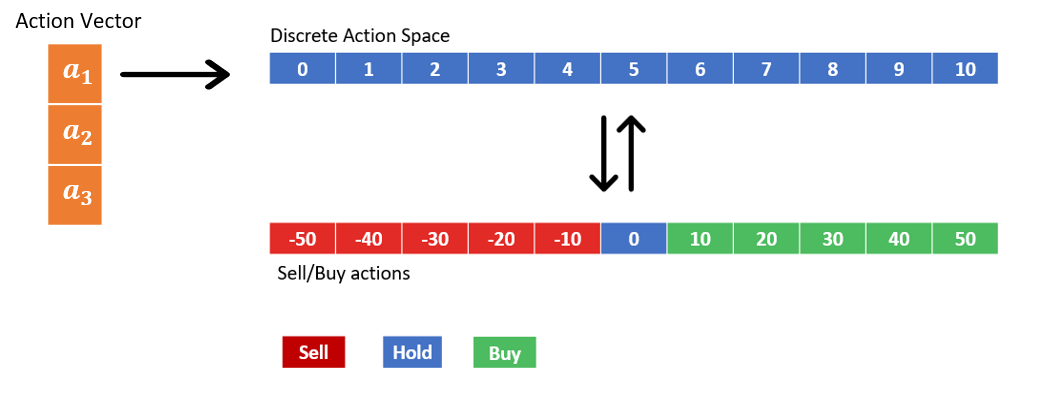The observation space has a dictionary structure with two elements. These represent the current trading state and the current action mask values. The trading state is a 7×1 vector consisting of the quantities of each assets currently held by the agent, current cash balance, and the current market value of each of the three assets. The action mask is a 3×11 matrix with mask values corresponding to each possible action. The environment calculates the mask values at every time using an `update_mask()` method. Actions that violate any of the constraints C1:C4 are assigned a zero mask. The value of mask is set to be 1 for admissible actions. See the following code:

``````def update_mask(self): self.action_mask=[np.array([1.0]*x.n) for x in self.action_space.spaces] # Set all masks to 1 if self.balance<1: # If balance < 1, set buy masks to zero (C4) for jj in range(len(self.action_mask)): self.action_mask[jj][6:]=[0.0]*5 self.action_mask[6:]=[0.0]*5 if (self.prices*self.assets/self.total_assets)>1.0/3.0 else [1.0]*5 #(C3) self.action_mask[6:]=[0.0]*5 if (self.prices*self.assets/self.total_assets)>2.0/3.0 else [1.0]*5 #(C2) for k in range(3): cap=int(min(5,self.assets[k]/10)) self.action_mask[k][:5]=[0.0]*(5-cap)+[1.0]*cap # (C1)
``````

At the beginning of each episode, a `reset()` method is called to reinitialize the trading state, observations, and other parameters. The agent starts each training episode with \$1,000 USD in cash balance and zero holdings in assets. Each episode consists of 20 investment periods.

`````` def reset(self): self.assets=np.zeros(3,dtype=np.float32) # Assets owned at the beginning self.balance=1000 # Initial cash balance self.t_step=0 self.prices=[np.random.normal(mu,var) for mu,var in zip(self.mu,self.var)] # Sampling market prices for the assets self.state=np.hstack([self.assets, self.balance, self.prices]) # Initial state self.total_assets=self.balance # Total portfolio value self.update_mask() # Updating action mask values reset_state={ "action_mask":list(np.float32(self.action_mask)), # Initial state "trading_state":np.float32(self.state) } return reset_state
``````

At the beginning of every investment period, the agent samples an action based on the latest observations it recorded and updates its portfolio. This is modeled using a `step()` method. After the portfolio is updated, we recalculate the state. The action mask is also updated by calling the `update_mask()` method.

``````def step(self, action): self.t_step+=1 for index, a in enumerate (action): print("action is ",a) print("price is ",self.prices[index]) quant=abs(a-5) # Number of assets traded/10 if a<5: # Condition: Asset sale ? if 10*quant*self.sell_price[index]>self.balance: # Condition: sale cost > Balance ? quant=np.floor(self.balance/(10*self.sell_price[index])) self.assets[index]-=10*quant # Asset update self.balance=self.balance+10*quant*(self.prices[index]-self.sell_price[index]) # Balance update if a>5: if 10*quant*(self.buy_price[index]+self.prices[index])>self.balance: # Condition: Buy cost > Balance ? quant=np.floor(self.balance/(10*(self.buy_price[index]+self.prices[index]))) self.assets[index]+=10*quant # Asset update self.balance=self.balance-10*quant*(self.prices[index]+self.sell_price[index]) # Balance update else: continue self.prices=np.array([np.random.normal(mu,var) for mu,var in zip(self.sig[:,self.t_step],self.var)]) # New asset prices self.state=np.hstack([self.assets,self.balance, self.prices]) # New state self.total_assets=self.balance+np.dot(self.assets,self.prices) # Total portfolio value self.update_mask() # Mask update obs={ "action_mask": list(np.float32(self.action_mask)), "trading_state":np.float32(self.state) } if self.t_step==len(self.tvec)-1: reward=self.total_assets # reward = Total portfolio value at the end of the episode else: reward=0 done=True if self.t_step==len(self.tvec)-1 else False return obs, reward, done, {}
``````

The reward function is defined as the final total portfolio value and calculated at the end of each episode, which happens after 20 investment periods.

At each time step, the environment returns the dictionary state and the ML model representing the policy samples an action based on this state. A parametric action model facilitates sampling only the unmasked (mask ≠ 0) actions. Here we describe the parametric actions model that enables action masking:

``````class ParametricActionsModel(TFModelV2): def __init__(self, obs_space, action_space, num_outputs, model_config, name, *args, **kwargs): super(ParametricActionsModel, self).__init__(obs_space, action_space, num_outputs, model_config, name, *args, **kwargs) self.true_obs_shape = (7,) self.action_embed_model = FullyConnectedNetwork(Box(np.finfo(np.float32).min,np.finfo(np.float32).max,shape=self.true_obs_shape), action_space, num_outputs, model_config, name, ) # action embedding model self.register_variables(self.action_embed_model.variables()) def forward(self, input_dict, state, seq_lens): action_mask= tf.cast(tf.concat(input_dict["obs"]["action_mask"], axis=1), tf.float32) # action mask values action_embedding,_ = self.action_embed_model({"obs":input_dict["obs"]["trading_state"]}) # action embeddings logit_mod = tf.maximum(tf.math.log(action_mask),tf.float32.min) # moidfiers to action logits return (action_embedding+logit_mod), state def value_function(self): return self.action_embed_model.value_function()
``````

Actions are sampled by the model through a Softmax function using the logits given by an action embedding model. This model is defined in the `__init__` method. The masking behavior itself is implemented in the `forward()` method. Here, we separate the actions masks and trading state from the dictionary state retrieved from the environment. The action embeddings are then obtained by passing the trading state to the action embedding network. Next, we modify the value of embeddings of each action by adding `logit_mod` to the logits. Notice that `logit_mod` is a function of the logarithm of the action mask. For actions with mask =1, the logarithm of mask will be zero, which leaves their embeddings unperturbed. On the other hand, when mask=0, the logarithm of mask → −∞. Because `Softmax(x)` →0 as x→ −∞, this makes sure that masked actions aren’t sampled by the agent.

Let’s test if the mask is working as expected. We initiate a ray trainer object and mask some of the actions and see if the trainer is sampling only the unmasked actions:

``````import ray
import ray.rllib.agents.ppo as ppo
from ray.tune.registry import register_env
ray.shutdown()
ray.init(ignore_reinit_error=True) env_config={}
state=env.reset()

The output in the following screenshot shows the initial action mask array.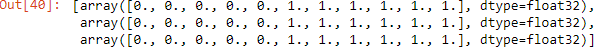Now we modify the mask vectors so that for a1, all choices except action 8 (buy 30 units of Asset 1); for a2 everything except action 5 (hold Asset 2 at current numbers); and for a3, everything except actions 1 and 2 (sell 40 or 30 units of Asset 3) are masked:

``````state["action_mask"]=[np.zeros(,dtype=np.float32) for _ in range(3)]
``````

Now that we have modified the action mask array, we try and sample a new action.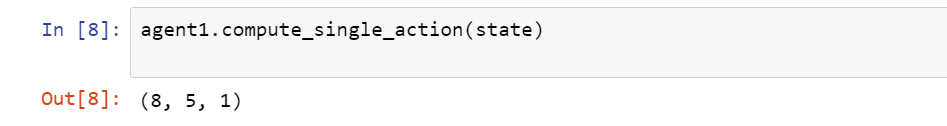The agent samples only those actions that are unmasked. This verifies that action masking is working as expected.

## Results

Now that the environment and parametric actions model are defined, we train an agent to solve the portfolio optimization problem using SageMaker RL. We train an RL agent to learn the optimal policy to maximize the reward under the constraints C1:C4. We use the proximal policy optimization (PPO) algorithm in SageMaker RL to train the RL agent for 500,000 episodes. The following training configuration shows how we specify the agent to use the `trading_mask` as a `custom_model` to be used:

`` def get_experiment_config(self): return { "training": { "env": "mytradingmodel", "run": "PPO", # Use PPO algorithm "stop":{"episodes_total":500000}, # 500k training episodes "config": { "use_pytorch": False, "gamma": 0.99, "kl_coeff": 1.0, "num_sgd_iter": 20, "lr": 0.0001, "sgd_minibatch_size": 1000, "train_batch_size": 25000, "monitor": True, "model": { "custom_model": "trading_mask" # Use custom action masking model }, "num_workers": (self.num_cpus-1), "num_gpus": self.num_gpus, "batch_mode": "truncate_episodes", "explore":True, "exploration_config":{ "type":"StochasticSampling", }, }, "checkpoint_freq": 1, } }``

The agent starts with \$1,000 USD in initial cash balance. The mean reward per episode is plotted as a function of training time, as shown in the following chart. Recall that we use the final total portfolio value as reward. At the end of 20 investment periods, we observe that the mean value of the agent’s portfolio is over \$3,000 USD.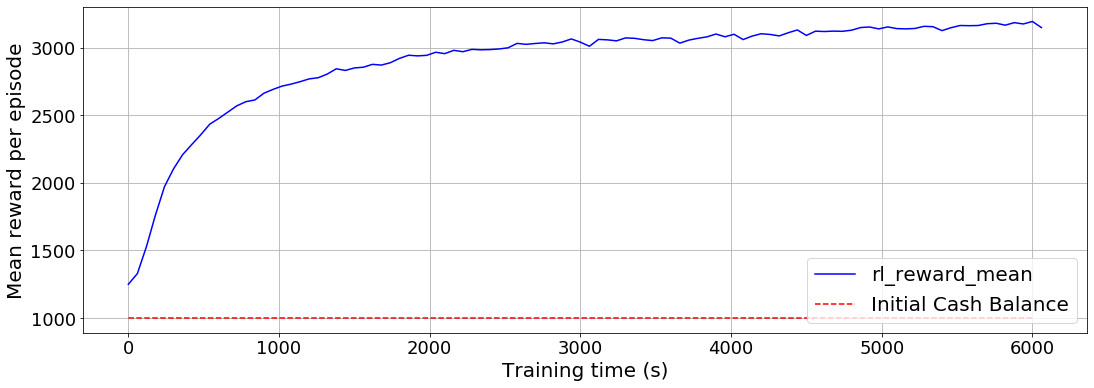## Clean up

We didn’t provision any infrastructure beyond the use of a SageMaker notebook instance. If you’re using a SageMaker notebook instance via Studio, you can shut it down by following the instructions in Shut Down an Open Notebook.

## Conclusion

In this post, we discussed how you can implement action masking to enforce constraints in RL model training. By masking inadmissible actions, we enable the agent to sample only valid actions and learn the optimal policy in a sample efficient manner. We introduced a portfolio optimization problem wherein the agent is tasked with maximizing their portfolio value by trading three asset types under multiple constraints. We demonstrated how to implement multi-dimensional action masking for this problem using Ray RLlib. We trained an RL agent for solving the constrained portfolio optimization problem using SageMaker RL.

Now that you know how to perform action masking using SageMaker RL and Ray RLlib on portfolio optimization, you can try it on other RL problems that involve inadmissible actions. You can also adapt the action masking code developed in this post for simpler problems involving one-dimensional action space. We encourage you to apply the approach developed here to your RL use cases and let us know if you have any questions or feedback.Dilshad Raihan Akkam Veettil is a Data Scientist with AWS Professional Services, where he engages with customers across industries to solve their business challenges through the use of machine learning and cloud computing. He holds a PhD in Aerospace Engineering from Texas A&M University, College Station. In his leisure time, he enjoys watching football and reading.Paul Budnarain is an Applied Scientist in Amazon’s Inventory Forecasting Systems (IFS) group, and is based out of Los Angeles,California.